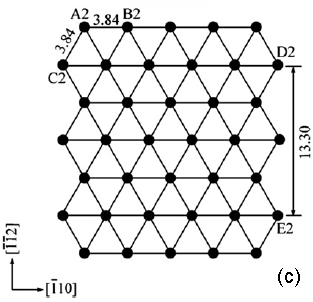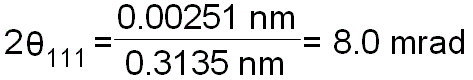Diffraction Patterns & Crystallography of Silicon (Si)
- Practical Electron Microscopy and Database -
- An Online Book -Microanalysis | EM Book                                                                   https://www.globalsino.com/EM/

Some examples of kinematically forbidden reflections in silicon (Si) are:
i) {222} reflections.
ii) ±(002) reflections in the  orientation. In thick TEM specimen those reflections arise due to double scattering (double diffraction) by the ±(-111) and ±(1-11) reflections. They are also induced partially by the fact that the “geometrical part” of the Lorentz factor  may enhance their intensities since the four {111} reflections reflect the largest net-plane spacing in any <110> projection.

Figure 4489 shows the arrangement of atoms on Si (111).Figure 4489. Arrangement of atoms on Si (111).

For crystals, the angles between the direct beam and diffraction spots can be calculated. For instance, the wavelength of 200-kV TEM is 0.00251 nm, and d111 for Si is 0.3135 nm, thus the angle between direct beam and Si 111 spot is given by (see page3580),------------------- [4489a]

Crystal Si has cubic structure. Its lattice parameter is 0.543 nm so that the (111) lattice spacing (see page3547) can be given by,------------------- [4489b]

Table 4489 lists the angles (2θB) between the direct beam 000 and diffracted beams as well as the lattice spacings for Si at accelerating voltages of 200, 300 and 400 kV. The angles can be computed with the DM scripts in the table.

 Table 4489. Angles (2θB) between the direct beam 000 and diffracted beams as well as the lattice spacings for a Si crystal (a = 5.431195(9) Å).

 Moeck, P.; Rouvimov, S.: Structural fingerprinting of nanocrystals in the transmission electron microscope: utilizing information on projected reciprocal lattice geometry, 2D symmetry, and structure factors. In: Drug Delivery Nanoparticles Formulation and Characterization (Eds. Y. Pathak, D. Thassu), Informa, 2009 (Drugs and the Pharmaceutical Sciences, Vol. 191), pp. 270–313, (ISBN-13: 978-1-4200-7804-6).

 ;